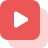Home » About GATE » GATE study materials » Mechanical Engineering » Introduction to Heisler Charts

# Introduction to Heisler Charts

Heisler charts are a graphical analytic tool used in thermal engineering to evaluate one-dimensional transient conduction heat flow.

Heisler charts are graphical analytic tools used in thermal engineering to evaluate heat transmission. They are a collection of two charts per contained geometry developed in 1947 by M. P. Heisler and expanded in 1961 by H. Gröber with a triple chart per geometry.

For example, the centre temperature of transient heat conduction through an indefinitely long flat wall of thickness 2L, an endlessly long cylinder of radius ro, as well as a sphere of radius ro, may be calculated using Heisler charts. In this article, we will discuss the concept of Heisler chart heat transfer.

### How does the Heisler Charts calculator operate?

The original Heisler-Gröber charts were built on the first term of an infinite planar wall’s accurate Fourier Series solution:

[T (x, t) −T∞]/[Ti−T∞]=∑ [(4sinλn/(2λn+sin2λn) e-λ2n at/L2 * cos λnx/L]

Ti is the slab’s starting temperature, T∞ is the boundary’s constant temperature, x is the plane wall’s position, λn is π(n+1/2), and a is thermal diffusivity. The location x=0 represents the slab’s centre.

The dependency of dimensionless temperature on time is the same throughout a particular shape. This implies that even if the dimensionless centre temperatures drop by 10% at a given period, the dimensionless temperature everywhere else in the geometry falls by 10%. This reliance generates ratios used to construct the y-axis of most Heisler charts.

For example, when conduction and convection resistances are nearly equal, and Biot no is 1, the Heisler charts calculator determines temperature distribution and heat flow rate. The ratio of internal conductive resistance to exterior convective resistance is known as the Biot number.

### Biot and Fourier Numbers

The Biot & Fourier numbers are two dimensionless quantities that may be used to represent temperature distribution and heat flows:

Internal-conduction resistance is minimal in contrast to surface-convection resistance for all values of the Bi number. Therefore, all values of Fo no. indicate that the body must be heated or cooled for an extended length of time.

### Charts

The plane wall’s initial chart is made up of three different factors. The dimensionless temperature at the midplane is plotted along the chart’s vertical axis. The Fourier number is plotted along the horizontal axis, Fo = αt/L2.

Finally, the curves in the graph are a collection of values for the Biot number’s inverse. Its heat transfer coefficient is h, and k is the material’s thermal conductivity.

For various Biot values, the second chart is being used to estimate the fluctuation in temperature inside the plane wall at some other locations there in the x-direction at the same time.

The vertical axis shows the ratio of a specific temperature to being at the centerline, in which the x/L curve denotes the point at which T is measured.

The value of Bi1 is represented on the horizontal axis.

Gröber supplied the third chart in each set in 1961, and this one depicts the dimensionless heat transmitted from the wall as a function of a non – dimensional time variable.

A plot of Q/Qo, the proportion of actual heat transmission to total feasible heat transfer until T = T∞, is shown on the vertical axis. The (Bi2) (Fo) graph, a non – dimensional time variable, is on the horizontal axis.

### How to use Heisler Charts?

There are several alternative methods to use these charts, included below.

They should compute the Fourier’s number to get the x-axis coordinate and the required temperature if the time is supplied. Then, the Biot number’s reverse should be solved to decide which red curves to employ. The value of θ0 /θi, or the temperature ratios on the y-axis, is returned when the x-axis position and the curve cross. This value is used to determine the temperature at a particular time.

Suppose the existing, initial, and final temperatures are known, and the duration where the current temperature occurs is required. In that case, the procedure is the same as described above, except that rather than calculating the Fourier’s number to solve for temperature ratio, the temperature ratio, as well as inverse Biot number, must be calculated first to count the Fourier’s number, that can then be reorganised to solve for the time.

### Conclusion

Heisler charts are a graphical tool used in thermal engineering to evaluate one-dimensional transient conduction heat flow. They are a collection of two charts per included geometry introduced in 1947 by M. P. Heisler and expanded in 1961 by H. Gröber with the third chart per geometry. The central temperature for transient heat conduction through an indefinitely long flat wall of thickness 2L, an endlessly long cylinder of radius ro, and a sphere of the radius may be calculated using Heisler charts. Three charts depicting the midplane temperature, temperature distribution, and heat transport may be used to study each of the geometries mentioned above.Get answers to the most common queries related to the GATE Examination Preparation.

## What is the contribution of control volume in fluid flow?

Ans : A control-volume analysis proves to be useful in fluid flow as it is mainly concentrated on a...Read full

## How to choose the direction of the control-volume?

Ans : Choosing an easier control-volume is always the priority. A rectangle that has the XY axes, can have an unknown amount of fl...Read full